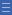# Fraction fiddle

Fraction fiddle

The digital learning object illustrates the relationship between the part-whole model of a fraction and the value of the fraction, by representing its position on a number line.

Display the learning object L2800 Fraction fiddle: tool to the class on an interactive whiteboard (or projector and screen).

• Enter a fraction (e.g. $$\frac{1}{3}$$) and discuss how a bar can be constructed to represent that fraction.
• Point out that the length of the bar is the same as the distance between 0 and 1 on the number line, and so helps to locate the fraction on the number line. The fraction bar acts as a bridge from the typical area model for representing fractions to a linear model.
• Select another fraction (e.g. $$\frac{1}{4}$$) and ask students to predict what the bar diagram will look like, and where the fraction will be located on the number line. Ask questions such as:
• Will $$\frac{1}{4}$$be smaller or larger than $$\frac{1}{3}$$?
• Will $$\frac{1}{4}$$ be closer to 1 than $$\frac{1}{3}$$?
• Emphasise the fact that, together, the numerator and denominator form a number that has a value, and therefore a place on the number line.

Yes

Yes

Name Class SectionYear 3: Model and represent unit fractions including 1/2, 1/4, 1/3, 1/5 and their multiples to a complete whole Infobox 3Year 4: Count by quarters halves and thirds, including with mixed numerals. Locate and represent these fractions on a number line Infobox 3Year 5: Compare and order common unit fractions and locate and represent them on a number line Infobox 3Source Infobox 3